﻿ 一维Boussinesq方程反问题与正问题的联合求解 The United Solution for Positive and Inverse Problems of One-Dimensional Boussinesq Equation

Pure Mathematics
Vol. 08  No. 06 ( 2018 ), Article ID: 27523 , 6 pages
10.12677/PM.2018.86087

The United Solution for Positive and Inverse Problems of One-Dimensional Boussinesq Equation

Yuhong Jin, Leihao Zuo*

Department of Basic Courses, Naval University of Engineering, Wuhan HubeiReceived: Oct. 19th, 2018; accepted: Oct. 31st, 2018; published: Nov. 13th, 2018ABSTRACT

In this paper, the inverse problem and the positive problem of the one-dimensional Boussinesq equation are solved jointly by using the finite difference method. In the solving process, the nonlinear least square method is used for optimization. The approximate solution is consistent with the truth value.

Keywords:Inverse Problems, Boussinesq Equation, Finite Difference Method, Nonlinear Least Square Method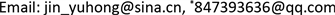1. 反问题的提出

$\left\{\begin{array}{l}\frac{\partial h}{\partial t}=\frac{\partial }{\partial x}\left[k\left(x\right)h\left(x,t\right)\frac{\partial h}{\partial x}\right]+f\left(x,t\right)\\ h\left(x,0\right)={\phi }_{1}\left(x\right)\\ h\left(0,t\right)={g}_{1}\left(t\right)\\ h\left(1,t\right)={g}_{2}\left(t\right)\\ 0\le x\le 1,0\le t\le 1\end{array}$

2. 有限差分法

2.1. 差分格式的建立

$\left\{\begin{array}{l}\frac{\partial h}{\partial t}=\frac{\partial }{\partial x}\left[k\left(x\right)h\left(x,t\right)\frac{\partial h}{\partial x}\right]+f\left(x,t\right)\\ h\left(x,0\right)={\phi }_{1}\left(x\right)\\ h\left(0,t\right)={g}_{1}\left(t\right)\\ h\left(1,t\right)={g}_{2}\left(t\right)\\ 0\le x\le 1,0\le t\le 1\end{array}$

$\frac{\partial }{\partial x}\left[k\left(x\right)h\left(x,t\right)\frac{\partial h}{\partial x}\right]=\frac{\partial k\left(x\right)}{\partial x}\ast h\left(x,t\right)\ast \frac{\partial h}{\partial x}+k\left(x\right)\ast \frac{\partial h\left(x,t\right)}{\partial x}\ast \frac{\partial h}{\partial x}+k\left(x\right)\ast h\left(x,t\right)\ast \frac{{\partial }^{2}h}{\partial {x}^{2}}$

$\left\{\begin{array}{l}{{h}^{\prime }}_{t}\left({x}_{i},{t}_{0}\right)=\frac{1}{2\tau }\left(-3h\left({x}_{i},{t}_{0}\right)+4h\left({x}_{i},{t}_{1}\right)-h\left({x}_{i},{t}_{2}\right)\right)\\ {{h}^{\prime }}_{t}\left({x}_{i},{t}_{j}\right)=\frac{1}{2\tau }\left(-h\left({x}_{i},{t}_{j-1}\right)+h\left({x}_{i},{t}_{j+1}\right)\right),\text{\hspace{0.17em}}0

$\frac{\partial k\left(x\right)}{\partial x}$ 离散化后的三点数值微分公式表示为：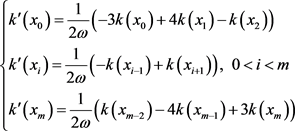$\frac{\partial h}{\partial x}$ 离散化后的三点数值微分公式：

$\left\{\begin{array}{l}{{h}^{\prime }}_{x}\left({x}_{0},{t}_{j}\right)=\frac{1}{2\omega }\left(-3h\left({x}_{0},{t}_{j}\right)+4h\left({x}_{1},{t}_{j}\right)-h\left({x}_{2},{t}_{j}\right)\right)\\ {{h}^{\prime }}_{x}\left({x}_{i},{t}_{j}\right)=\frac{1}{2\omega }\left(-h\left({x}_{j-1},{t}_{j}\right)+h\left({x}_{j+1},{t}_{j}\right)\right),\text{\hspace{0.17em}}\text{\hspace{0.17em}}0

$\frac{{\partial }^{2}h}{\partial {x}^{2}}$ 离散化后的三点数值微分公式：

$\left\{\begin{array}{l}{{h}^{″}}_{x}\left({x}_{0},{t}_{j}\right)=\frac{1}{{\omega }^{2}}\left(h\left({x}_{2},{t}_{j}\right)-2h\left({x}_{1},{t}_{j}\right)+h\left({x}_{0},{t}_{j}\right)\right)\\ {{h}^{″}}_{x}\left({x}_{i},{t}_{j}\right)=\frac{1}{{\omega }^{2}}\left(h\left({x}_{i+1},{t}_{j}\right)-2h\left({x}_{i},{t}_{j}\right)+h\left({x}_{i-1},{t}_{j}\right)\right),\text{\hspace{0.17em}}\text{\hspace{0.17em}}0

2.2. 方程组求解

3. 数值实验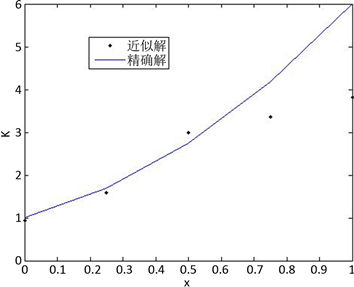Figure 1. $m=5,n=5$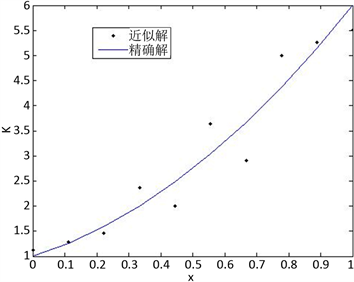Figure 2. $m=10,n=10$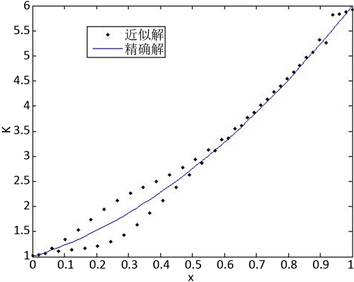Figure 3. $m=50,n=50$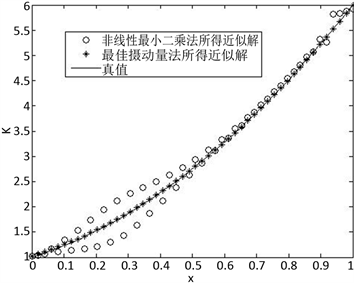Figure 4. ComparisonTable 1. Average residual sum of squares under different grid densities

The United Solution for Positive and Inverse Problems of One-Dimensional Boussinesq Equation[J]. 理论数学, 2018, 08(06): 650-655. https://doi.org/10.12677/PM.2018.86087

1. 1. 田娜. 偏微分方程反问题数值解研究与应用[D]: [博士学位论文]. 无锡: 江南大学, 2012.

2. 2. 王兵贤, 徐定华, 胡康秀. 一维流动的Boussinesq方程渗流系数反演的变分伴随方法研究[J]. 数学的实践与认识, 2008, 38(20): 194-200.

3. 3. 王兵贤, 王泽文, 徐定华, 胡康秀. 二维流Boussinesq方程渗透系数反演的变分伴随方法[J]. 水利水电科技进展, 2010, 30(6): 11-14.

4. 4. 张海丽. 非线性抛物型方程若干类反问题的研究[D]: [硕士学位论文]. 杭州: 浙江理工大学, 2010.

5. 5. 潘树龙, 孙维夫. 抛物型偏微分方程的多步有限差分法计算方法[J]. 烟台职业学院学报, 2011, 17(1): 76-80.

6. 6. 刘春风, 彭亚绵. 偏微分方程并行算法及反问题数值解法[M]. 北京: 清华大学出版社, 2015: 183-185.